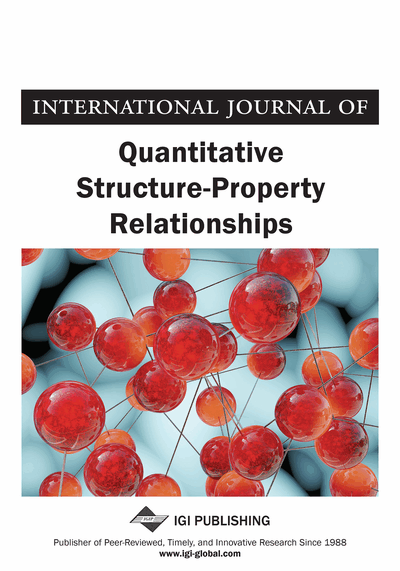# QSPR-Modeling for the Second Virial Cross-Coefficients of Binary Organic Mixtures

Elena Mokshyna (O.V. Bogatsky Physico-Chemical Intstitute NAS of Ukraine, Odessa, Ukraine), Pavel Polishchuk (O.V. Bogatsky Physico-Chemical Intstitute NAS of Ukraine, Odessa, Ukraine), Vadim Nedostup (O.V. Bogatsky Physico-Chemical Intstitute NAS of Ukraine, Odessa, Ukraine) and Victor Kuz'min (O.V. Bogatsky Physico-Chemical Intstitute NAS of Ukraine, Odessa, Ukraine)
DOI: 10.4018/IJQSPR.2016070104
Available
\$37.50
No Current Special Offers

## Abstract

The second virial cross-coefficient is an important characteristic of the pair intermolecular interactions that describes solely the heterogeneous interactions. In the current study, the authors made the first attempt to develop rigorous QSPR models for analysis and prediction of the second virial cross-coefficient. Novel descriptors to describe pair intermolecular interactions were implemented. Statistical characteristics of the obtained models showed high performance. Prediction errors are comparable to the errors of data. Theoretically predicted values of the second virial cross-coefficient may be used to derive PVT-properties of mixtures at the different temperatures as well as to calculate intermolecular pair potential.
Article Preview
Top

## Introduction

The virial equitation of state (1) is the only equation of state that gives a precise theoretical description for a system of interacting particles (Moran, 2000):

(1)

In Equation (1), p is the pressure, ρ is the density that equals to 1/V, T is the thermodynamic temperature, R is the universal gas constant, B and C are the second one and the third one virial coefficients correspondingly.

The virial equation allows to calculate the pVT properties with high precision at low and moderate pressures (J. H. Dymond, K. Marsh, R. Wilhoit, & K. Wong, 2002). The coefficients are rigorously connected with the interaction energy. B is connected with the energy of pair interactions, C is connected with the energy of triple interactions, etc. The Equation (1) is strictly applicable towards gas mixtures due to accurate dependency of the virial coefficients on a composition of mixture. For the second virial coefficient, this dependency has the following form (Dymond, 2002):

(2)

In Equation (2), x is the mole fraction of compounds 1 and 2; B1 and B2 are the second virial coefficients of pure compounds, and B12 –is the second virial cross-coefficient.

The second virial cross-coefficient is a calculated property defined purely by pair heterogeneous interactions of mixture’s components. Unlike the other properties of mixtures, the second virial cross coefficient characterizes only an interaction of molecule 1 with molecule 2. Because properties of mixtures mainly depend on the pair interactions, the second virial cross-coefficient gives a possibility to predict pVT properties not only for binary mixtures, but also for multicomponent mixtures.

Previously the present group of authors (Mokshyna et al., 2015) obtained QSPR models for the second virial coefficient of pure compounds with predictive accuracy close to the errors of data. The aim of the current study is the development of QSPR methodology for B12 (Equation 2) prediction.

Existing methods of prediction for the second virial coefficient use the corresponding states principle (i.e., require additional experimental data) and do not show high predictive accuracy for various organic compounds (Poling, Prausnitz, O'Connell, 2001). There is a wide potential use of easy and fast prediction method for the second virial coefficient – from theoretical calculations of the intermolecular interaction potential to industrial calculations for the technological processes. In the current study, authors developed the ensemble of models able to describe and predict the second virial cross-coefficients for binary organic mixtures. As far as we know, no attempts to build QSPR models for the second virial cross-coefficient were yet made, therefore this study is the first one.

## Complete Article List

Search this Journal:
Reset
Open Access Articles
Volume 7: 4 Issues (2022): 1 Released, 3 Forthcoming
Volume 6: 4 Issues (2021)
Volume 5: 4 Issues (2020)
Volume 4: 4 Issues (2019)
Volume 3: 2 Issues (2018)
Volume 2: 2 Issues (2017)
Volume 1: 2 Issues (2016)
View Complete Journal Contents Listing